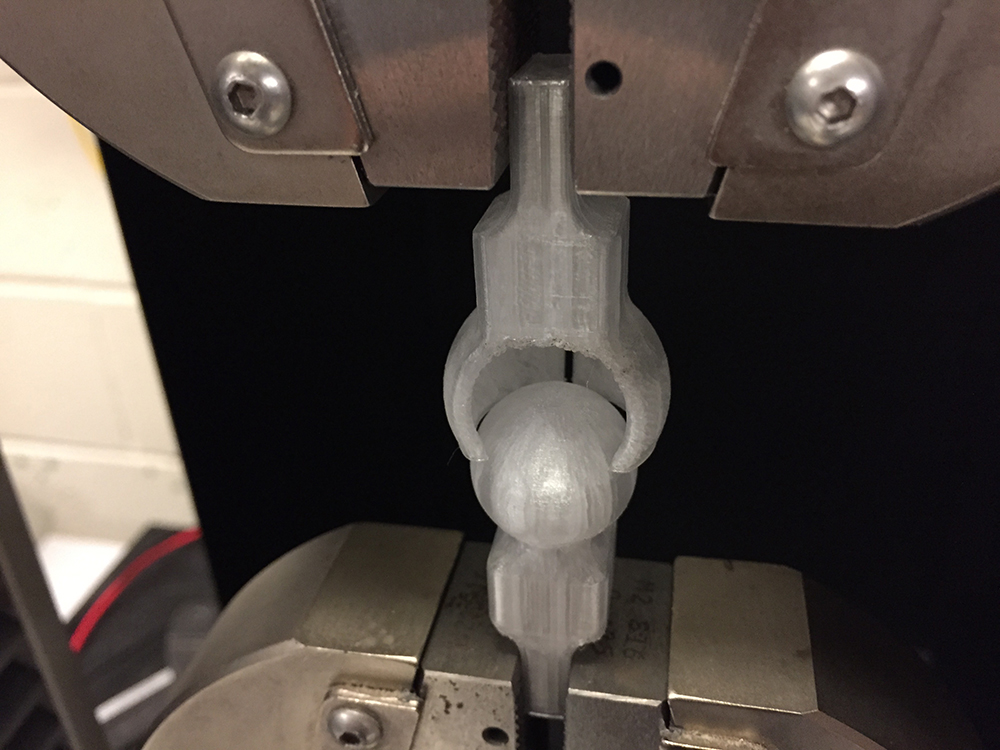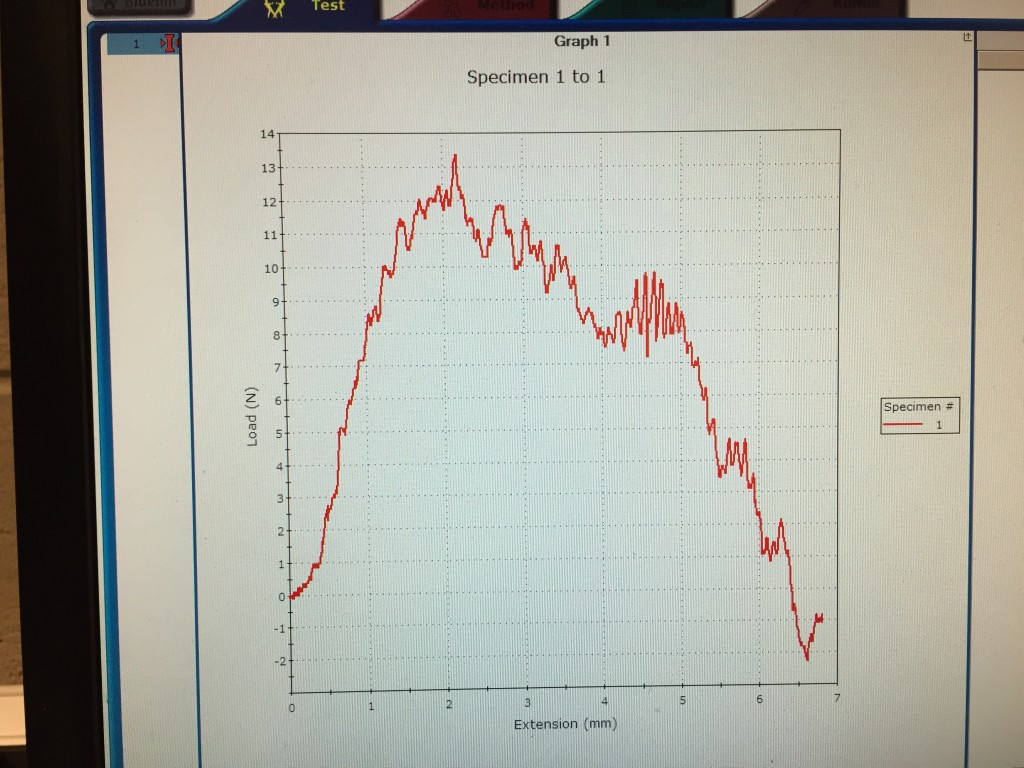Skip to ContentDan k
Chen
Material Stress Strain Study
Explorations Dan Chen / March 3, 2016

Measure the stiffness and strength of a structure, and relate it to the material properties.

## Structure / Material: PET

My goal was to design a flexible join out of 3D printed material, PET. It should be strong enough to hold a certain weight and at the same time easy to take apart, assembled and reassembled.

I designed the model in SolidWorks, with one part shape like a sphere and the other like a cup.

To make the Instron clamps work with my design I shape the ends of my pieces into a quarter inch thick block, for easy grabbing.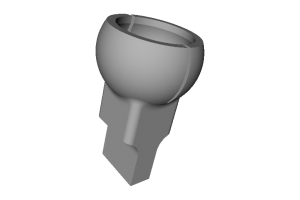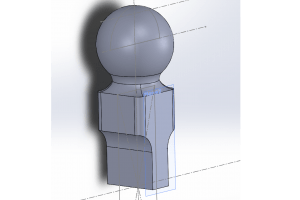## Fabrication & Tweaking

After several tests, tweaking the slot depth and the opening size. I found one sets of number that works well for me. It can be assembled with the hands pressing it together without breaking, and it won’t break apart.

Each part takes about an hour to print. In order to make the structure stick to the plate better, I made the bottom side flat.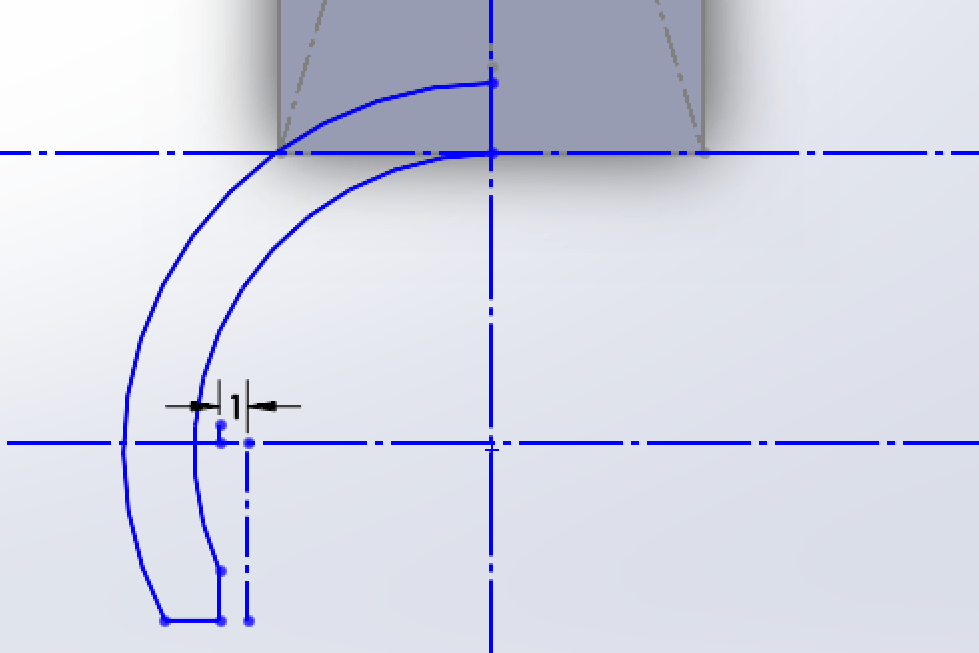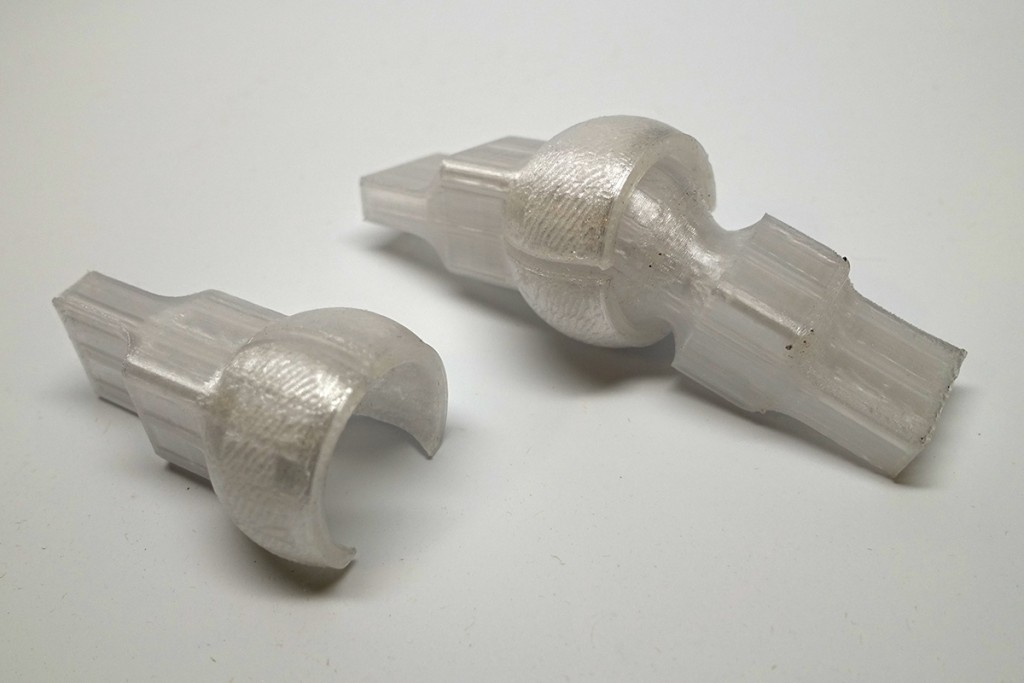## The Test SetupThe setup was very easy since I have the quarter inch handle of the clamps.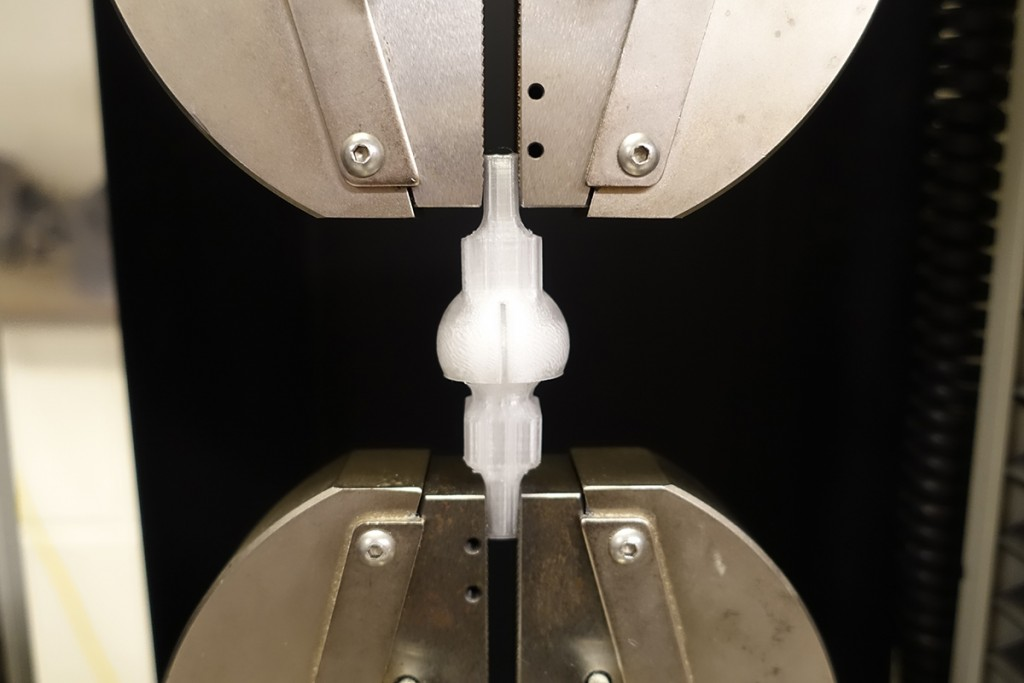http://youtu.be/4QwHzBUFL_Y

## Data Collection X3 ( time / mm / N )

The machine pulls and pushes the structure 3 times to produce the following graph. As you can see the data is pretty consistent.## Result: Tensile,  Compressive Stress & Young’s modulus

Stress = Force/Cross-sectional Area

Failure Force/Cross Sectional Area = Failure Stress or pull apart force
Strain = change in length/original length

Young’s (elastic) Modulus = stress/strain
or
E = stress / strain

Interactive Graph (Jquery Plot)

There are 2 events that I want to calculate theTensile Modulus (Young’s Modulus, Modulus of Elasticity) E

Pull

E = stress / strain

stress = 30/ (10 x 10mm)
strain = 12.5mm/10mm

E=0.3/1.25 = 0.24
Graph BelowPush

stress = 30/ (10 x 6mm)
strain = 10mm/6mm
E=0.5/1.66 = 0.30
Graph Below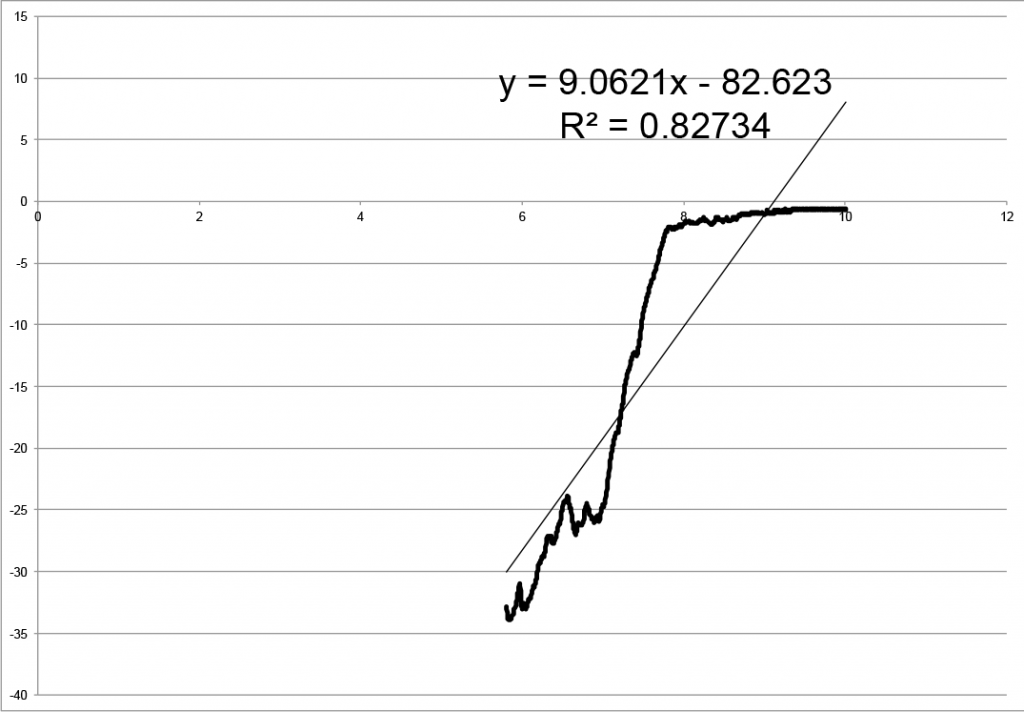Calculation example

## Material properties v.s. Structure

E=0.24  (Pull)
E=0.30  (Push)
Material’PET
E= 2

Both are within the Modulus of Elasticity of the material
Assuming that the 20% filled PET gives only 20% of the E.

2*0.2=0.4

0.4 is still grater than 0.24 (pull E) and 0.3 (push E)## Extra Test on Different Structure

With one side of the “cup” open, we see decrease in holding strength. 30N v.s. 13N